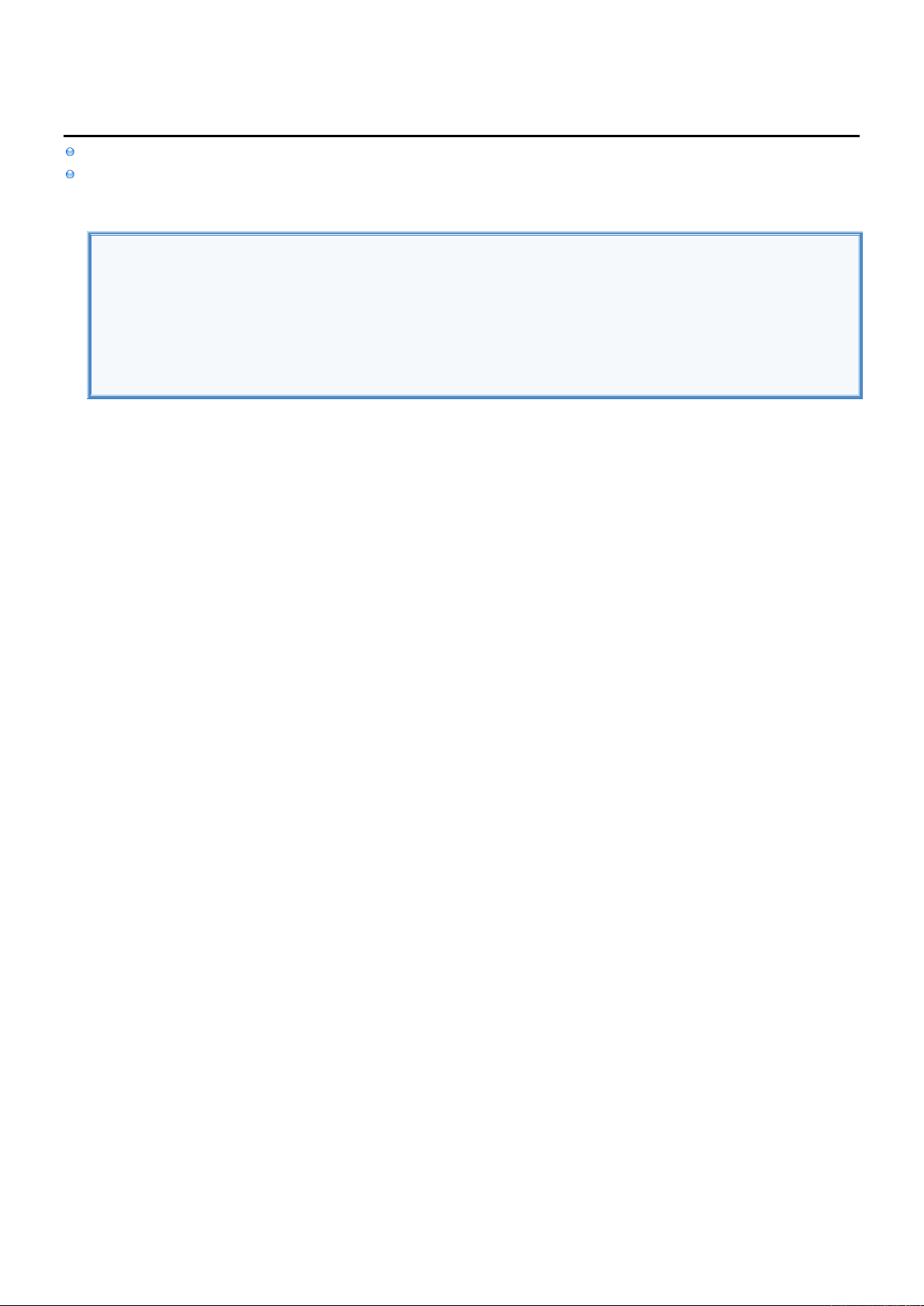2
2
.
.
1
1
.
.
2
2
P
P
r
r
i
i
n
n
t
t
t
t
o
o
C
C
o
o
n
n
s
s
o
o
l
l
e
e
I
I
n
n
f
f
o
o
To print to Console you can use print() and dump() Functions.
Inside the String you can reference Properties by using \(name).
Example
//DECLARE VARIABLES.
var name = "John"
var age = 20
//DISPLAY VARIABLES.
print("Hello " + name) //Hello John
print("\(name) is \(age) years old") //John is 20 years old
dump ("\(name) is \(age) years old") //John is 20 years old